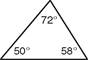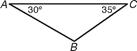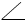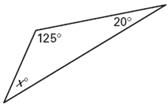Finding the 3rd Angle in a Triangle

If you add all three interior angle measures together in a triangle it will always equal 180°.. To find a third angle you will subtract the sum of the two given angles from 180Â°. Look at the 3 examples below.72° + 50° + 58° = 180°103° + 47° + 30° = 180°90° + 36° + 54° = 180°

Now let's look at what to do when we are given 2 angles but we are missing the 3rd angle.

 Step 1: add the two angles that you are given together Step 2: subtract your answer from 180

Example 1:Step 1: 30° + 35° = 65° Step 2: 180° - 65° = 115° mB = 115°

Example 2:Step 1: 90° + 20° = 110° Step 2: 180° - 110° = 70° mF = 70°

Example 3:Step 1: 125° + 20° = 145° Step 2: 180° - 145° = 35° x° = 35

The key to finding the missing angle measure of a triangle is to remember that the sum of the interior angles of any triangle is always 180°. If you know 2 angles then you can subtract their sum from 180° to find the measure of the 3rd angle.

 Related Links: Math Geometry Topics Classifying Triangles by Angles

To link to this Finding the 3rd Angle in a Triangle page, copy the following code to your site: